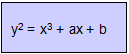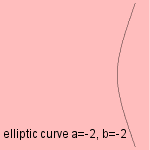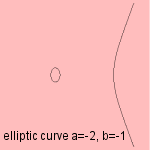# elliptic(al) curve

## cubicIt is possible to define these divergent parabolas as a type of cubic curve which is topologically equivalent to a torus.
The curves emerged at the studies of the elliptic integrals. There is also a recent application in code theory, where they are used to construct error repairing codes.
Quite recently the elliptic curves have been used for cryptography in so 'called elliptic curve cryptosystems'. But in 1997, when a first standard (named P1363) for this method just has been proposed, it appeared that some elliptic curves could be tracked down quite easily because of symmetry reasons (called 'trace one').

For the curve, either parameter a, either parameter b needs a value unequal zero.

Some examples: